nLab topology

Contents

This page is about topology as a field of mathematics. For topology as a structure on a set, see topological space.

Parts of this page exists also in a German language version, see at Topologie.

Contents

Idea

Topology is one of the basic fields of mathematics. The term is also used for a particular structure in a topological space; see topological structure for that.

The subject of topology deals with the expressions of continuity and boundary, and studying the geometric properties of (originally: metric) spaces and relations of subspaces, which do not change under continuous deformations, regardless to other (such as in their metric properties).

Topology as a structure enables one to model continuity and convergence locally. More recently, in metric spaces, topologists and geometric group theorists started looking at asymptotic properties at large, which are in some sense dual to the standard topological structure and are usually referred to as coarse topology.

There are many cousins of the concept of topological spaces, e.g. sites, locales, topoi, higher topoi, uniformity spaces and so on, which specialize or generalize some aspect or structure usually found in Top.

One of the tools of topology, homotopy theory, has long since crossed the boundaries of topology and applies to many other areas, thanks to many examples and motivations as well as of abstract categorical frameworks for homotopy like Quillen model categories, Brown’s categories of fibrant objects and so on.

Introduction

The following gives a quick introduction to some of the core concepts and tools of topology:

A detailed introduction is going to be at Introduction to Topology.

Continuity

The key idea of topology is to study spaces with “continuous maps” between them. The concept of continuity was made precise first in analysis, in terms of epsilontic analysis of open balls, recalled as def. below. Then it was realized that this has a more elegant formulation in terms of the more general concept of open sets, this is prop. below. Adopting the latter as the definition leads to the concept of topological spaces, def. below.

First recall the basic concepts from analysis:

Definition

(metric space)

A metric space is

1. a set $X$ (the “underlying set”);

2. a function $d \;\colon\; X \times X \to [0,\infty)$ (the “distance function”) from the Cartesian product of the set with itself to the non-negative real numbers

such that for all $x,y,z \in X$:

1. $d(x,y) = 0 \;\;\Leftrightarrow\;\; x = y$

2. (symmetry) $d(x,y) = d(y,x)$

3. (triangle inequality) $d(x,y)+ d(y,z) \geq d(x,z)$.

Example

Every normed vector space $(V, {\vert - \vert})$ becomes a metric space according to def. by setting

$d(x,y) \coloneqq {\vert x-y \vert} \,.$
Definition

(epsilontic definition of continuity)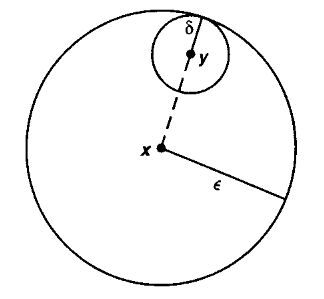For $(X,d_X)$ and $(Y,d_Y)$ two metric spaces (def. ), then a function

$f \;\colon\; X \longrightarrow Y$

is said to be continuous at a point $x \in X$ if for every $\epsilon \gt 0$ there exists $\delta\gt 0$ such that

$d_X(x,y) \lt \delta \;\;\Rightarrow\;\; d_Y(f(x), f(y)) \lt \epsilon$

or equivalently such that

$f(\;B_x^\circ(\delta)\;) \;\subset\; B^\circ_{f(x)}(\epsilon)$

where $B^\circ$ denotes the open ball (definition ).

The function $f$ is called just continuous if it is continuous at every point $x \in X$.

We now reformulate this analytic concept in terms of the simple but important concept of open sets:

Definition

(open ball)

Let $(X,d)$, be a metric space. Then for every element $x \in X$ and every $\epsilon \in \mathbb{R}_+$ a positive real number, write

$B^\circ_x(\epsilon) \;\coloneqq\; \left\{ y \in X \;\vert\; d(x,y) \lt \epsilon \right\}$

for the open ball of radius $\epsilon$ around $x$.

Definition

(neighbourhood and open set)

Let $(X,d)$ be a metric space (def. ). Say that

1. A neighbourhood of a point $x \in X$ is a subset $x \in U \subset X$ which contains some open ball $B_x^\circ(\epsilon)$ around $x$ (def. ).

2. An open subset of $X$ is a subset $U \subset X$ such that for every $x \in U$ it also contains a neighbourhood of $x$.

The following picture shows a point $x$, some open balls $B_i$ containing it, and two of its neighbourhoods $U_i$: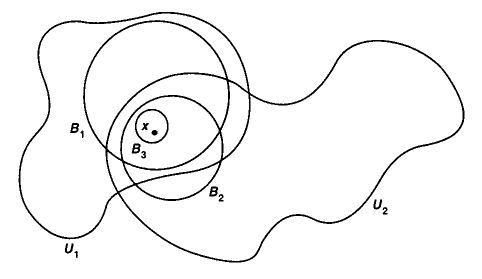graphics grabbed from Munkres 75

Proposition

(rephrasing continuity in terms of open sets)

A function $f \colon X \to Y$ between metric spaces (def. ) is continuous in the epsilontic sense of def. precisely if it has the property that its pre-images of open subsets of $Y$ (in the sense of def. ) are open subsets of $X$.

principle of continuity

$\,$ $\,$ Pre-Images of open subsets are open.

Proof

First assume that $f$ is continuous in the epsilontic sense. Then for $O_Y \subset Y$ any open subset and $x \in f^{-1}(O_Y)$ any point in the pre-image, we need to show that there exists a neighbourhood of $x$ in $f^{-1}(O_Y)$. But by assumption there exists an open ball $B_x^\circ(\epsilon)$ with $f(B_x^\circ(\epsilon)) \subset O_Y$. Since this is true for all $x$, by definition this means that $f^{-1}(O_Y)$ is open in $X$.

Conversely, assume that $f^{-1}$ takes open subsets to open subsets. Then for every $x \in X$ and $B_{f(x)}^\circ(\epsilon)$ an open ball around its image, we need to produce an open ball $B_x^\circ(\delta)$ in its pre-image. But by assumption $f^{-1}(B_{f(x)}^\circ(\epsilon))$ contains a neighbourhood of $x$ which by definition means that it contains such an open ball around $x$.

Topological spaces

Therefore we should pay attention to open subsets. It turns out that the following closure property is what characterizes the concept:

Proposition

(closure properties of open sets in a metric space)

The collection of open subsets of a metric space $(X,d)$ as in def. has the following properties:

1. The intersection of any finite number of open subsets is again an open subset.

2. The union of any set of open subsets is again an open subset.

In particular

• the empty set is open (being the union of no subsets)

and

• the whole set $X$ itself is open (being the intersection of no subsets).

This motivates the following generalized definition:

Definition

(topological spaces)

Given a set $X$, then a topology on $X$ is a collection $\tau$ of subsets of $X$ called the open subsets, hence a subset of the power set

$\tau \subset P(X)$

such that this is closed under forming

1. finite intersections;

2. arbitrary unions.

A topological space is a set $X$ equipped with such a topology.

The following shows all the topologies on the 3-element set (up to permutation of elements)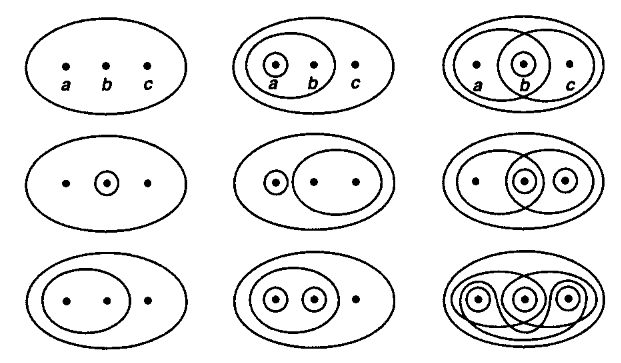graphics grabbed from Munkres 75

It is now immediate to formally implement the

principle of continuity

$\,$ $\,$ Pre-Images of open subsets are open.

Definition

(continuous maps)

$f \colon (X, \tau_X) \to (Y, \tau_Y)$

is a function between the underlying sets,

$f \colon X \longrightarrow Y$

such that pre-images under $f$ of open subsets of $Y$ are open subsets of $X$.

The simple definition of open subsets and the simple principle of continuity gives topology its fundamental and universal flavor. The combinatorial nature of these definitions makes topology closely related to formal logic (for more on this see at locale).

# Remark

(the category of topological spaces)

The composition of continuous functions is clearly associative and unital.

One says that

1. topological spaces constitute the objects

2. continuous maps constitute the morphisms (homomorphisms)

of a category. The category of topological spaces (“Top” for short).

It is useful to depict collections of objects with morphisms between them by diagrams, like this one: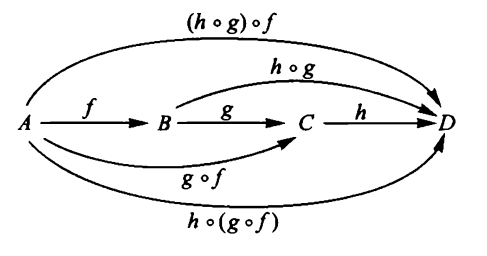graphics grabbed from Lawvere-Schanuel 09.

Our motivating example now reads:

Example

(metric topology)

Let $(X,d)$ be a metric space. Then the collection of open subsets in def. constitutes a topology on the set $X$, making it a topological space in the sense of def. . This is called the metric topology.

Stated more concisely: the open balls in a metric space constitute a “basis” for the metric topology.

One point of the general definition of “topological space” is that it admits constructions which intuitively should exist on “continuous spaces”, but which do not in general exist, for instance, as metric spaces:

Example

(discrete topological space)

For $S$ a bare set, then the discrete topology on $S$ regards every subset of $S$ as an open subset.

Example

(subspace topology)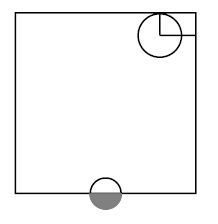Let $(X, \tau_X)$ be a topological space, and let $X_0 \hookrightarrow X$ be a subset of the underlying set. Then the corresponding topological subspace has $X_0$ as its underlying set, and its open subsets are those subsets of $X_0$ which arise as restrictions of open subsets of $X$.

(Also called the initial topology of the inclusion map.)

The picture on the right shows two open subsets inside the square, regarded as a topological subspace of the plane $\mathbb{R}^2$:

graphics grabbed from Munkres 75

Example

(quotient topological space)

Let $(X,\tau_X)$ be a topological space (def. ) and let

$R_\sim \subset X \times X$

be an equivalence relation on its underlying set. Then the quotient topological space has

and

• a subset $O \subset X_{\sim}$ is declared to be an open subset precisely if its preimage $\pi^{-1}(O)$ under the canonical projection map

$\pi \colon X \to X_\sim$

is open in $X$.

(This is also called the final topology of the projection $\pi$.)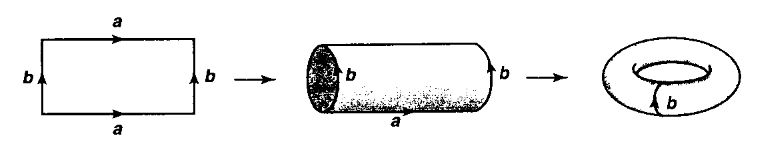The above picture shows on the left the square (a topological subspace of the plane), then in the middle the resulting quotient topological space obtained by identifying two opposite sides (the cylinder), and on the right the further quotient obtained by identifying the remaining sides (the torus).

graphics grabbed from Munkres 75

Example

(product topological space)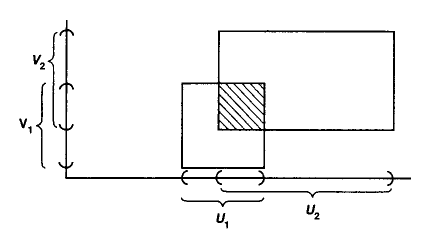For $X$ and $Y$ two topological spaces, then the product topological space $X \times Y$ has

• as underlying set the Cartesian product of the underlying sets of $X$ and $Y$,

and

• its open sets are those subsets $O \subset X \times Y$ of the Cartesian product such that for all point $(x,y) \in O$ there exists open sets $x \in O_x \subset X$ and $y \in O_Y \subset Y$ such that $O_x \times O_y \subset O$.

graphics grabbed from Munkres 75

These constructions of discrete topological spaces, quotient topological spaces, topological subspaces and of product topological spaces are simple examples of limits and of colimits of topological spaces. The category Top of topological spaces has the convenient property that all limits and colimits (over small diagrams) exist in it. (For more on this see at Top – Universal constructions.)

Homeomorphism

With the objects (topological spaces) and the morphisms (continuous maps) of the category Top of topology thus defined, we obtain the concept of “sameness” in topology.

To make this precise, one says that a morphism

$X \overset{f}{\to} Y$

in a category is an isomorphism if there exists a morphism going the other way around

$X \overset{f^{-1}}{\longleftarrow} Y$

which is an inverse in the sense that

$f \circ f^{-1} = id_Y \;\;\;\;\; and \;\;\;\;\; f^{-1} \circ f = id_X \,.$
Definition

(homeomorphisms)

An isomorphism in the category Top of topological spaces with continuous functions between them is called a homeomorphism.

Hence this is a continuous function

$f \;\colon\; X \longrightarrow Y$

such that there exists an inverse morphism, namely a continuous function the other way around

$X \longleftarrow Y \;\colon\; f^{-1}$

such that

$f \circ f^{-1} = id_{Y} \;\;\;and\;\;\; f^{-1} \circ f = id_{X} \,.$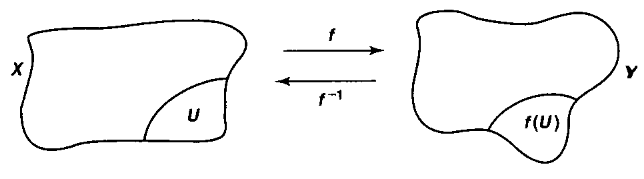graphics grabbed from Munkres 75

Example

(open interval homeomorphic to the real line)

The open interval $(-1,1)$ is homeomorphic to all of the real line

$(0,1) \underset{homeo}{\simeq} \mathbb{R}^1 \,.$

An inverse pair of continuous functions is for instance given by

$\array{ f &\colon& \mathbb{R}^1 &\longrightarrow& (-1,+1) \\ && x &\mapsto& \frac{x}{\sqrt{1+ x^2}} }$

and

$\array{ f^{-1} &\colon& (-1,+1) &\longrightarrow& \mathbb{R}^1 \\ && x &\mapsto& \frac{x}{\sqrt{1 - x^2}} } \,.$

Generally, every open ball in $\mathbb{R}^n$ (def. ) is homeomorphic to all of $\mathbb{R}^n$.

Example

(interval glued at endpoints is homeomorphic to the circle)

As topological spaces, the interval with its two endpoints identified is homeomorphic (def. ) to the standard circle:

$[0,1]_{/(0 \sim 1)} \;\; \underset{homeo}{\simeq} \;\; S^1 \,.$

More in detail: let

$S^1 \hookrightarrow \mathbb{R}^2$

be the unit circle in the plane

$S^1 = \{(x,y) \in \mathbb{R}^2, x^2 + y^2 = 1\}$

equipped with the subspace topology (example ) of the plane $\mathbb{R}^2$, which itself equipped with its standard metric topology (example ).

Moreover, let

$[0,1]_{/(0 \sim 1)}$

be the quotient topological space (example ) obtained from the interval $[0,1] \subset \mathbb{R}^1$ with its subspace topology by applying the equivalence relation which identifies the two endpoints (and nothing else).

Consider then the function

$f \;\colon\; [0,1] \longrightarrow S^1$

given by

$t \mapsto (cos(t), sin(t)) \,.$

This has the property that $f(0) = f(1)$, so that it descends to the quotient topological space

$\array{ [0,1] &\overset{}{\longrightarrow}& [0,1]_{/(0 \sim 1)} \\ & {}_{\mathllap{f}}\searrow & \downarrow^{\mathrlap{\tilde f}} \\ && S^1 } \,.$

We claim that $\tilde f$ is a homeomorphism (definition ).

First of all it is immediate that $\tilde f$ is a continuous function. This follows immediately from the fact that $f$ is a continuous function and by definition of the quotient topology (example ).

So we need to check that $\tilde f$ has a continuous inverse function. Clearly the restriction of $f$ itself to the open interval $(0,1)$ has a continuous inverse. It fails to have a continuous inverse on $[0,1)$ and on $(0,1]$ and fails to have an inverse at all on [0,1], due to the fact that $f(0) = f(1)$. But the relation quotiented out in $[0,1]_{/(0 \sim 1)}$ is exactly such as to fix this failure.

Similarly:

The square $[0,1]^2$ with two of its sides identified is the cylinder, and with also the other two sides identified is the torus: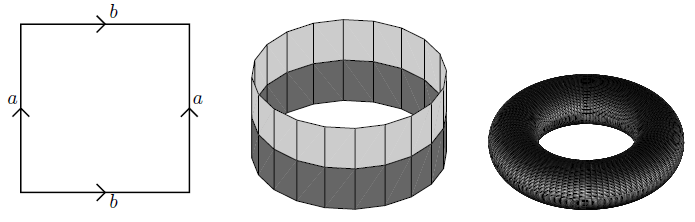If the sides are identified with opposite orientation, the result is the Möbius strip: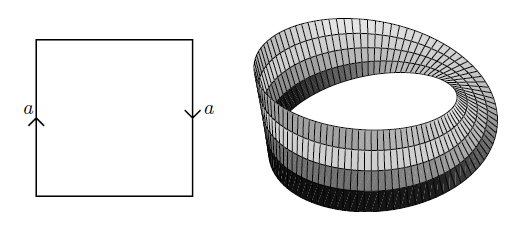graphics grabbed from Lawson 03

$\,$

Important examples of pairs of spaces that are not homeomorphic include the following:

# Theorem

(topological invariance of dimension)

For $n_1, n_2 \in \mathbb{N}$ but $n_1 \neq n_2$, then the Cartesian spaces $\mathbb{R}^{n_1}$ and $\mathbb{R}^{n_2}$ are not homeomorphic.

More generally, an open set in $\mathbb{R}^{n_1}$ is never homeomorphic to an open set in $\mathbb{R}^{n_2}$ if $n_1 \neq n_2$.

The proof of theorem is surprisingly hard, given how obvious the statement seems intuitively. It requires tools from a field called algebraic topology (notably Brouwer's fixed point theorem).

We showcase some basic tools of algebraic topology now and demonstrate the nature of their usage by proving two very simple special cases of the topological invariance of dimension (prop. and prop. below).

Example

(homeomorphism classes of surfaces)

The 2-sphere $S^2 = \{(x,y,z) \in \mathbb{R}^3 \vert x^2 + y^2 + z^2 = 1\}$ is not homeomorphic to the torus $T^2 = S^1 \times S^1$.

Generally the homeomorphism class of a closed orientable surface is determined by the number of “holes” it has, its genus.

$\,$

Homotopy

We have seen above that for $n \geq 1$ then the open ball $B_0^\circ(1)$ in $\mathbb{R}^n$ is not homeomorphic to, notably, the point $\ast = \mathbb{R}^0$ (example , theorem ). Nevertheless, intuitively the $n$-ball is a “continuous deformation” of the point, obtained as the radius of the $n$-ball tends to zero.

This intuition is made precise by observing that there is a continuous function out of the product topological space (example ) of the open ball with the closed interval

$\eta \colon [0,1] \times B_0^\circ(1) \longrightarrow \mathbb{R}^n$

which is given by rescaling:

$(t,x) \mapsto t \cdot x \,.$

This continuously interpolates between the open ball and the point in that for $t = 1$ then it restricts to the defining inclusion $B_0^\circ(1)$, while for $t = 0$ then it restricts to the map constant on the origin.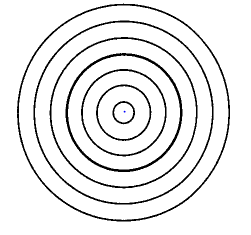We may summarize this situation by saying that there is a diagram of continuous functions of the form

$\array{ B_0^\circ(1) \times \{0\} \\ \downarrow & \searrow^{\mathrlap{x \mapsto 0}} \\ [0,1] \times B_0^\circ(1) &\overset{(t,x) \mapsto t \cdot x}{\longrightarrow}& \mathbb{R}^n \\ \uparrow & \nearrow_{\mathrlap{inclusion}} \\ B_0^\circ(1) \times \{1\} }$

Such “continuous deformations” are called homotopies:

Definition

(homotopy)

For $f,g\colon X \longrightarrow Y$ two continuous functions between topological spaces $X,Y$, then a (left) homotopy

$\eta \colon f \,\Rightarrow_L\, g$
$\eta \;\colon\; X \times I \longrightarrow Y$

out of the product topological space (example ) of the open ball with the standard interval, such that this fits into a commuting diagram of the form$\array{ {0} \times X \\ {}^{\mathllap{(id,\delta_0)}}\downarrow & \searrow^{\mathrlap{f}} \\ [0,1] \times X &\stackrel{\eta}{\longrightarrow}& Y \\ {}^{\mathllap{(id,\delta_1)}}\uparrow & \nearrow_{\mathrlap{g}} \\ \{1\} \times X } \,.$

graphics grabbed from J. Tauber here

Definition

(homotopy equivalence)

A continuous function $f \;\colon\; X \longrightarrow Y$ is called a homotopy equivalence if

1. there exists a continuous function the other way around, $g \;\colon\; Y \longrightarrow X$, and

2. left homotopies, def. , from the two composites to the identity:

$\eta_1 \;\colon\; f\circ g \Rightarrow_L id_Y$

and

$\eta_2 \;\colon\; g\circ f \Rightarrow_L id_X \,.$
Example

(open ball is contractible)

Any open ball (or closed ball), hence (by example ) any Cartesian space is homotopy equivalent to the point

$\mathbb{R}^n \underset{homotopy}{\simeq} \ast \,.$
Example

The following three graphs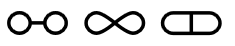(i.e. the evident topological subspaces of the plane $\mathbb{R}^2$ that these pictures indicate) are not homeomorphic. But they are homotopy equivalent, in fact they are each homotopy equivalent to the disk with two points removed, by the homotopies indicated by the following pictures: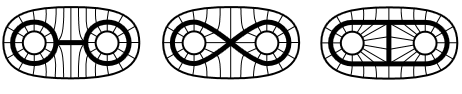graphics grabbed from Hatcher

$\,$

Connected components

Using the concept of homotopy one obtains the basic tool of algebraic topology, namely the construction of algebraic homotopy invariants of topological spaces. We introduce the simplest and indicate their use.

Example

A homotopy between two points

$x,y \;\colon\; \ast \to X$

is a continuous path between these points.

Definition

(connected components)

The set of homotopy classes of points in a topological space $X$ is called its set of path-connected components, denoted.

$\pi_0(X) \in Set \,.$

If $\pi_0(X) \simeq \ast$ consists of a single element, we say that $X$ is a path-connected topological space.

If $f \colon X \to Y$ is a continuous map between topological spaces, then on homotopy classes of points it induces a function between the corresponding connected components, which we denote by:

$\pi_0(f) \;\colon\; \pi_0(X) \longrightarrow \pi_0(Y) \,.$

This construction is evidently compatible with composition, in that

$\pi_0(g \circ f) = \pi_0(g) \circ \pi_0(f)$

and it evidently is unital, in that

$\pi_0(id_X) = id_{\pi_{0}(X)} \,.$

One summarizes this by saying that $\pi_0$ is a functor from the category Top of topological spaces to the category Set of sets, denoted

$\pi_0 \;\colon\; Top \longrightarrow Set \,.$

An immediate but important consequence is this:

Proposition

If two topological spaces have sets of connected components that are not in bijection, then those spaces are not homeomorphic to each other:

$\pi_0(X) \neq \pi_0(Y) \;\;\; \Rightarrow \;\;\, X \underset{homeo}{\neq} Y$
Proof

Since $\pi_0$ is functorial, it immediately follows that it sends isomorphisms to isomorphisms, hence homeomorphisms to bijections:

\begin{aligned} & f \circ g = id \;\;and\;\; g \circ f = id \\ \Rightarrow \;\;\;\;\;\;& \pi_0(f \circ g) = \pi_0(id) \;\;and \;\; \pi_0(g \circ f) = \pi_0(id) \\ \Leftrightarrow \;\;\;\;\;\; & \pi_0(f) \circ \pi_0(g) = id \;\;and \;\; \pi_0(g) \circ \pi_0(f) = id \end{aligned} \,.

This means that we may use path connected components as a first “topological invariant” that allows us to distinguish some topological spaces.

principle of algebraic topology

$\,\,$ Use topological invariants to distinguish topological spaces.

As an example for how this is being used, we have the following proof of a simple special case of the topological invariance of dimension (theorem ):

Proposition

(topological invariance of dimension – first simple case)

The Cartesian spaces $\mathbb{R}^1$ and $\mathbb{R}^2$ are not homeomorphic (def. ).

Proof

Assume there were a homeomorphism

$f \colon \mathbb{R}^1 \longrightarrow \mathbb{R}^2$

we will derive a contradiction. If $f$ is a homeomorphism, then clearly so is its restriction to the topological subspaces (example ) obtained by removing $0 \in \mathbb{R}^1$ and $f(0) \in \mathbb{R}^2$.

$f \;\colon\; (\mathbb{R}^1-\{0\}) \longrightarrow (\mathbb{R}^2 - \{f(0)\}) \,.$

It follows that we would get a bijection of connected components between $\pi_0(\mathbb{R}^1 - \{0\})$ and $\pi_0(\mathbb{R}^2 - \{f(0)\})$. But clearly the first set has two elements, while the second has just one:

$\pi_0(\mathbb{R}^1-\{0\}) \;\neq\; \pi_0(\mathbb{R}^2 - \{f(0)\}) \,.$

The key lesson of the proof of prop. is its strategy:

principle of algebraic topology

$\,\,$ Use topological invariants to distinguish topological spaces.

Of course in practice one uses more sophisticated invariants than just $\pi_0$.

The next topological invariant after the connected components is the fundamental group:

Fundamental group

Definition

(fundamental group)

Let $X$ be a topological space and let $x \in X$ be a chosen point. Then write

$\pi_1(X,x) \;\in\; Grp$

for, to start with, the set of homotopy classes of paths in $X$ that start and end at $x$. Such paths are also called the continuous loops in $X$ based at $x$.

1. Under concatenation of loops, $\pi_1(X,x)$ becomes a semi-group.

2. The constant loop is a neutral element under this composition (thus making $\pi_1(X,x)$ a “monoid”).

3. The reverse of a loop is its inverse in $\pi_1(X,x)$, making $\pi_1(X,x)$ indeed into a group.

This is called the fundamental group of $X$ at $x$.

The following picture indicates the four non-equivalent non-trivial generators of the fundamental group of the oriented surface of genus 2: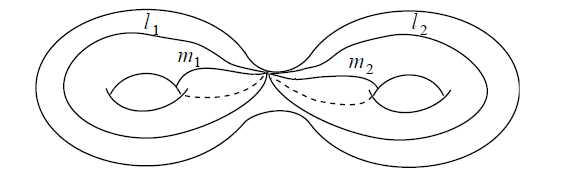graphics grabbed from Lawson 03

Again, this operation is functorial, now on the category $Top^{\ast/}$ of pointed topological spaces, whose objects are topological spaces equipped with a chosen point, and whose morphisms are continuous maps $f \colon X \to Y$ that take the chosen basepoint of $X$ to that of $Y$:

$\pi_1 \;\colon\; Top^{\ast/} \longrightarrow Grp \,.$

As $\pi_0$, so also $\pi_1$ is a topological invariant. As before, we may use this to prove a simple case of the theorem of the topological invariance of dimension:

Definition

A topological space $X$ for which

1. $\pi_0(X) \simeq \ast$ (path connected, def. )

2. $\pi_1(X,x) \simeq 1$ (the fundamental group is trivial, def. ),

is called simply connected.

Proposition

(topological invariance of dimension – second simple case)

There is no homeomorphism between $\mathbb{R}^2$ and $\mathbb{R}^3$.

Proof

Assume there were such a homeomorphism $f$; we will derive a contradiction.

If $f$ is a homeomorphism, then so is its restriction to removing the origin from $\mathbb{R}^2$ and $f(0)$ from $\mathbb{R}^3$:

$(\mathbb{R}^2 - \{0\}) \longrightarrow (\mathbb{R}^3 - \{f(0)\}) \,.$

Thse two spaces are both path-connected, hence $\pi_0$ does not distiguish them.

But they do have different fundamental groups $\pi_1$:

1. The fundamental group of $\mathbb{R}^{2} - \{0\}$ is $\mathbb{Z}$ (counting the winding of loops around the removed point). We discuss this further below in example .

2. The fundamental group of $\mathbb{R}^3 - \{f(0)\}$ is trivial: because the single removed point is no obstruction to sliding loops past it and contracting them.

But since passing to fundamental groups is functorial, the same argument as in the proof of prop. shows that $f$ cannot be an isomorphism, hence not a homeomorphism.

We now discuss a “dual incarnation” of fundamental groups, which often helps to compute them.

Covering spaces

Definition

(covering space)

A covering space of a topological space $X$ is a continuous map

$p \colon E \to X$

such that there exists an open cover $\underset{i}{\sqcup}U_i \to X$, such that restricted to each $U_i$ then $E \to X$ is homeomorphic over $U_i$ to the product topological space (example ) of $U_i$ with the discrete topological space (example ) on a set $F_i$

$\array{ \underset{i}{\sqcup} U_i \times F_i &\longrightarrow& E \\ \downarrow &(pb)& \downarrow^{\mathrlap{p}} \\ \underset{i}{\sqcup} U_i &\underset{}{\longrightarrow}& X } \,.$

For $x \in U_i \subset X$ a point, then the elements in $F_x = F_i$ are called the leaves of the covering at $x$.

Example

(covering of circle by circle)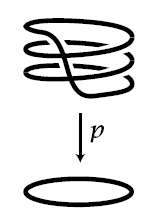Regard the circle $S^1 = \{ z \in \mathbb{C} \;\vert\; {\vert z\vert} = 1 \}$ as the topological subspace of elements of unit absolute value in the complex plane. For $k \in \mathbb{N}$, consider the continuous function

$p \coloneqq (-)^k \;\colon\; S^1 \longrightarrow S^1$

given by taking a complex number to its $k$th power. This may be thought of as the result of “winding the circle $k$ times around itself”. Precisely, for $k \geq 1$ this is a covering space (def. ) with $k$ leaves at each point.

graphics grabbed from Hatcher

Example

(covering of circle by real line)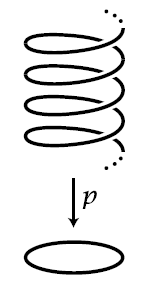Consider the continuous function

$\exp(2 \pi i(-)) \;\colon\; \mathbb{R}^1 \longrightarrow S^1$

from the real line to the circle, which,

1. with the circle regarded as the unit circle in the complex plane $\mathbb{C}$, is given by

$t \mapsto \exp(2\pi i t)$
2. with the circle regarded as the unit circle in $\mathbb{R}^2$, is given by

$t \mapsto ( cos(2\pi t), sin(2\pi t) ) \,.$

We may think of this as the result of “winding the line around the circle ad infinitum”. Precisely, this is a covering space (def. ) with the leaves at each point forming the set $\mathbb{Z}$ of natural numbers.

Definition

(action of fundamental group on fibers of covering)

Let $E \overset{\pi}{\longrightarrow} X$ be a covering space (def. )

Then for $x \in X$ any point, and any choice of element $e \in F_x$ of the leaf space over $x$, there is, up to homotopy, a unique way to lift a representative path in $X$ of an element $\gamma$ of the the fundamental group $\pi_1(X,x)$ (def. ) to a continuous path in $E$ that starts at $e$. This path necessarily ends at some (other) point $\rho_\gamma(e) \in F_x$ in the same fiber. This construction provides a function

$\array{ \rho &\colon& F_x \times \pi_1(X,x) &\longrightarrow& F_x \\ && (e,\gamma) &\mapsto& \rho_\gamma(e) }$

from the Cartesian product of the leaf space with the fundamental group. This function is compatible with the group-structure on $\pi_1(X,x)$, in that the following diagrams commute:

$\array{ F_x \times \{const_x\} && \longrightarrow && F_x \times \pi_1(X,x) \\ & {}_{\mathllap{id}}\searrow && \swarrow_{\mathrlap{\rho}} \\ && F_x } \;\;\;\;\;\; \left( \array{ \text{the neutral element,} \\ \text{i.e. the constant loop,} \\ \text{acts trivially} } \right)$

and

$\array{ F_x \times \pi_1(X,x) \times \pi_1(X,x) &\overset{\rho \times id}{\longrightarrow}& F_x \times \pi_1(X,x) \\ {}^{\mathllap{id \times ((-)\cdot(-))}}\downarrow && \downarrow^{\mathrlap{\rho}} \\ F_x \times \pi_1(X,x) &\underset{\rho}{\longrightarrow}& F_x } \;\;\;\;\;\; \left( \array{ \text{acting with two group elements } \\ \text{is the same as} \\ \text{first multiplying them} \\ \text{ and then acting with their product element} } \right) \,.$

One says that $\rho$ is an action or permutation representation of $\pi_1(X,x)$ on $F_x$.

For $G$ any group, then there is a category $G Set$ whose objects are sets equipped with an action of $G$, and whose morphisms are functions which respect these actions. The above construction yields a functor

$Cov(X) \longrightarrow \pi_1(X,x) Set \,.$
Example

(three-sheeted covers of the circle)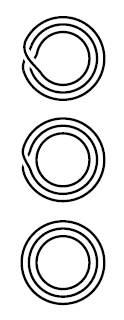There are, up to isomorphism, three different 3-sheeted covering spaces of the circle $S^1$.

The one from example for $k = 3$. Another one. And the trivial one. Their corresponding permutation actions may be seen from the pictures on the right.

graphics grabbed from Hatcher

We are now ready to state the main theorem about the fundamental group. Except that it does require the following slightly technical condition on the base topological space. This condition is satisfied for all “reasonable” topological spaces:

Definition

(semi-locally simply connected)

A topological space $X$ is called

1. locally path-connected if for every point $x \in X$ and for every neighbourhood $x \in U \subset X$ there exists a neighbourhood $x \in V \subset U$ such that $V$ is path-connected (def. );

2. semi-locally simply connected if every point $x \in X$ has a neighbourhood $x \in U \subset X$ such that the induced morphism of fundamental groups $\pi_1(U,x) \to \pi_1(X,x)$ is trivial (i.e. sends everything to the neutral element).

Theorem

(fundamental theorem of covering spaces)

Let $X$ be a topological space which is path-connected (def. ), locally path connected (def. ) and semi-locally simply connected (def. ). Then for any $x \in X$ the functor

$Fib_x \;\colon\; Cov(X) \overset{}{\longrightarrow} \pi_1(X,x) Set \,.$

from def. that describes the action of the fundamental group of $X$ on the set of leaves over $x$ has the following property:

1. every isomorphism class of $\pi_1(X,x)$-actions is in the image of the functor (one says: the functor is essentially surjective);

2. for any two covering spaces $E_1, E_2$ of $X$ then the map on morphism sets

$Fib_x \;\colon\; Hom_{Cov(X)}(E_1, E_2) \longrightarrow Hom( Fib_x(E_1), Fib_x(E_2) )$

is a bijection (one says the functor is a fully faithful functor ).

A functor with these two properties one calls an equivalence of categories:

$Cov(X) \overset{\simeq}{\longrightarrow} \pi_1(X,x) Set \,.$

This has some interesting implications:

Every sufficiently nice topological space $X$ as above has a covering which is simply connected (def. ). This is the covering corresponding, under the fundamental theorem of covering spaces (theorem ) to the action of $\pi_1(X)$ on itself. This is called the universal covering space $\hat X \to X$. The above theorem implies that the fundamental group itself may be recovered as the automorphisms of the universal covering space:

$\pi_1(X) \simeq Aut_{Cov_{/X}}(\hat X, \hat X) \,.$
Example

(computing the fundamental group of the circle)

The covering $\exp(2\pi i(-)) \;\colon\; \mathbb{R}^1 \to S^1$ from example is simply connected (def. ), hence must be the universal covering space, up to homeomorphism.

It is fairly straightforward to see that the only homeomorphisms from $\mathbb{R}^1$ to itself over $S^1$ are given by integer translations by $n \in \mathbb{N} \hookrightarrow \mathbb{R}$:

$\array{ \mathbb{R}^1 && \underoverset{\simeq}{t \mapsto t + n}{\longrightarrow} && \mathbb{R}^1 \\ & {}_{\mathllap{\exp(2 \pi i(-))}}\searrow && \swarrow_{\mathrlap{\exp(2 \pi i(-))}} \\ && S^1 } \,.$

Hence

$Aut_{Cov_{/S^1}}(\hat S^1, \hat S^1) \simeq \mathbb{Z}$

and hence the fundamental group of the circle is the additive group of integers:

$\pi_1(S^1) \simeq \mathbb{Z} \,.$

$\,$

$\,$

Related entries

The following is an (incomplete) list of available $n$Lab entries related to topology.

sphere

Computational Topology

Informal overview includes

A canonical compendium is

• Nicolas Bourbaki, chapter 1 Topological Structures of Elements of Mathematics III: General topology, Springer 1971, 1990

Introductory textbooks to topology include

• John KelleyGeneral Topology, Graduate Texts in Mathematics, Springer 1955

• James Munkres, Topology, Prentice Hall 1975 (2000)

• Terry Lawson, Topology: A Geometric Approach, Oxford University Press (2003) (pdf)

• Steven Vickers, Topology via Logic, Cambridge University Press (1989)